# Abel-Poisson summation method

(diff) ← Older revision | Latest revision (diff) | Newer revision → (diff)

One of the methods for summing Fourier series. The Fourier series of a functionis summable by the Abel–Poisson method at a pointto a numberifwhere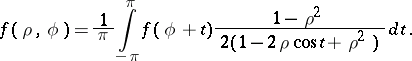(*)

If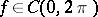, then the integral on the right-hand side is a harmonic function for, which is, as has been shown by S. Poisson, a solution of the Dirichlet problem for the disc. The Abel summation method applied to Fourier series was therefore named the Abel–Poisson summation method, and the integral (*) was named the Poisson integral.

If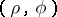are polar coordinates of a point inside the disc of radius one, then one can consider the limit ofas the point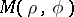approaches a point on the bounding circle not by a radial or by a tangential but rather along an arbitrary path. In this situation the Schwarz theorem applies: Ifbelongs to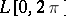and is continuous at a point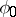, then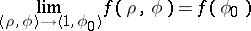irrespective of the path along which the pointapproaches the point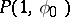as long as that path remains within the disc with radius one.

How to Cite This Entry:
Abel-Poisson summation method. Encyclopedia of Mathematics. URL: http://encyclopediaofmath.org/index.php?title=Abel-Poisson_summation_method&oldid=18630
This article was adapted from an original article by A.A. Zakharov (originator), which appeared in Encyclopedia of Mathematics - ISBN 1402006098. See original article